# INDEPENDENT SAMPLE T TEST.pptx

Study à University
1 Jun 2023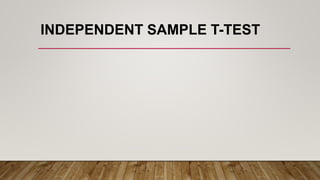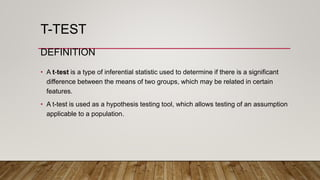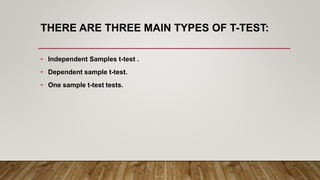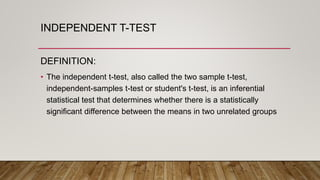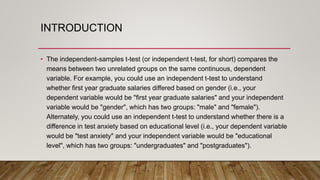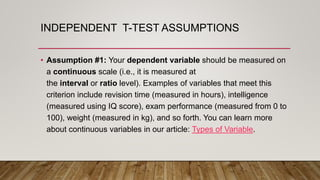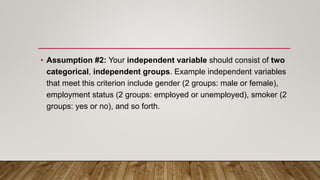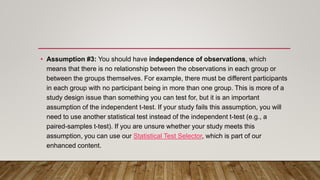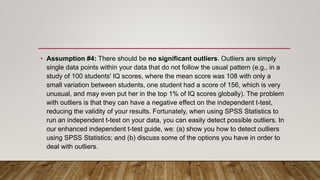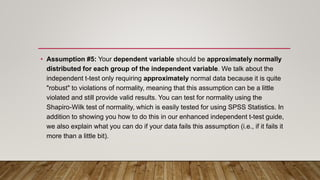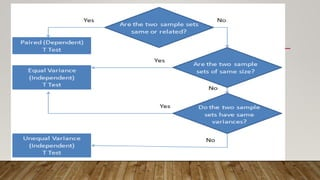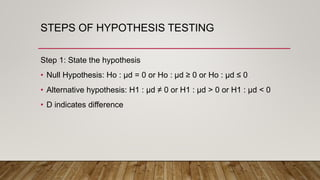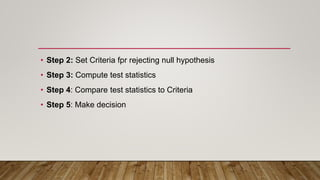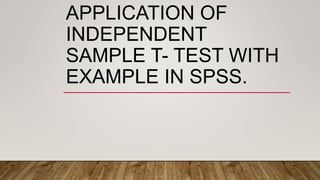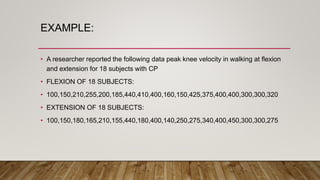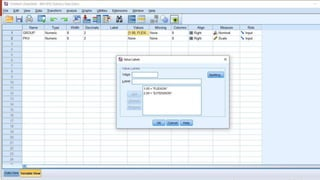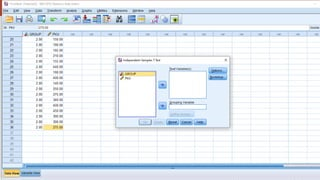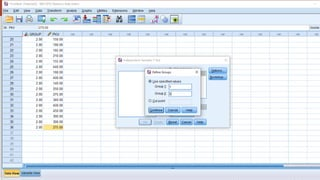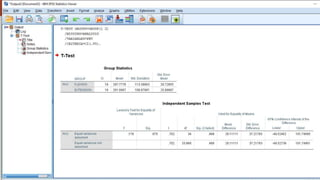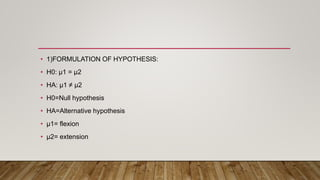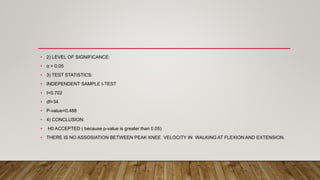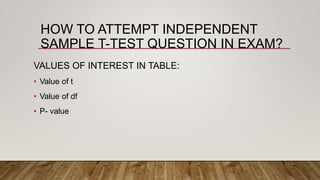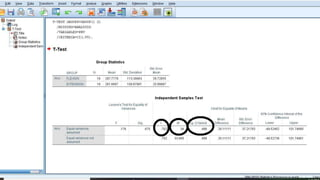1 sur 24

### INDEPENDENT SAMPLE T TEST.pptx

• 1. INDEPENDENT SAMPLE T-TEST
• 2. T-TEST DEFINITION • A t-test is a type of inferential statistic used to determine if there is a significant difference between the means of two groups, which may be related in certain features. • A t-test is used as a hypothesis testing tool, which allows testing of an assumption applicable to a population.
• 3. THERE ARE THREE MAIN TYPES OF T-TEST: • Independent Samples t-test . • Dependent sample t-test. • One sample t-test tests.
• 4. INDEPENDENT T-TEST DEFINITION: • The independent t-test, also called the two sample t-test, independent-samples t-test or student's t-test, is an inferential statistical test that determines whether there is a statistically significant difference between the means in two unrelated groups
• 5. INTRODUCTION • The independent-samples t-test (or independent t-test, for short) compares the means between two unrelated groups on the same continuous, dependent variable. For example, you could use an independent t-test to understand whether first year graduate salaries differed based on gender (i.e., your dependent variable would be "first year graduate salaries" and your independent variable would be "gender", which has two groups: "male" and "female"). Alternately, you could use an independent t-test to understand whether there is a difference in test anxiety based on educational level (i.e., your dependent variable would be "test anxiety" and your independent variable would be "educational level", which has two groups: "undergraduates" and "postgraduates").
• 6. INDEPENDENT T-TEST ASSUMPTIONS • Assumption #1: Your dependent variable should be measured on a continuous scale (i.e., it is measured at the interval or ratio level). Examples of variables that meet this criterion include revision time (measured in hours), intelligence (measured using IQ score), exam performance (measured from 0 to 100), weight (measured in kg), and so forth. You can learn more about continuous variables in our article: Types of Variable.
• 7. • Assumption #2: Your independent variable should consist of two categorical, independent groups. Example independent variables that meet this criterion include gender (2 groups: male or female), employment status (2 groups: employed or unemployed), smoker (2 groups: yes or no), and so forth.
• 8. • Assumption #3: You should have independence of observations, which means that there is no relationship between the observations in each group or between the groups themselves. For example, there must be different participants in each group with no participant being in more than one group. This is more of a study design issue than something you can test for, but it is an important assumption of the independent t-test. If your study fails this assumption, you will need to use another statistical test instead of the independent t-test (e.g., a paired-samples t-test). If you are unsure whether your study meets this assumption, you can use our Statistical Test Selector, which is part of our enhanced content.
• 9. • Assumption #4: There should be no significant outliers. Outliers are simply single data points within your data that do not follow the usual pattern (e.g., in a study of 100 students' IQ scores, where the mean score was 108 with only a small variation between students, one student had a score of 156, which is very unusual, and may even put her in the top 1% of IQ scores globally). The problem with outliers is that they can have a negative effect on the independent t-test, reducing the validity of your results. Fortunately, when using SPSS Statistics to run an independent t-test on your data, you can easily detect possible outliers. In our enhanced independent t-test guide, we: (a) show you how to detect outliers using SPSS Statistics; and (b) discuss some of the options you have in order to deal with outliers.
• 10. • Assumption #5: Your dependent variable should be approximately normally distributed for each group of the independent variable. We talk about the independent t-test only requiring approximately normal data because it is quite "robust" to violations of normality, meaning that this assumption can be a little violated and still provide valid results. You can test for normality using the Shapiro-Wilk test of normality, which is easily tested for using SPSS Statistics. In addition to showing you how to do this in our enhanced independent t-test guide, we also explain what you can do if your data fails this assumption (i.e., if it fails it more than a little bit).
• 12. STEPS OF HYPOTHESIS TESTING Step 1: State the hypothesis • Null Hypothesis: Ho : μd = 0 or Ho : μd ≥ 0 or Ho : μd ≤ 0 • Alternative hypothesis: H1 : μd ≠ 0 or H1 : μd > 0 or H1 : μd < 0 • D indicates difference
• 13. • Step 2: Set Criteria fpr rejecting null hypothesis • Step 3: Compute test statistics • Step 4: Compare test statistics to Criteria • Step 5: Make decision
• 14. APPLICATION OF INDEPENDENT SAMPLE T- TEST WITH EXAMPLE IN SPSS.
• 15. EXAMPLE: • A researcher reported the following data peak knee velocity in walking at flexion and extension for 18 subjects with CP • FLEXION OF 18 SUBJECTS: • 100,150,210,255,200,185,440,410,400,160,150,425,375,400,400,300,300,320 • EXTENSION OF 18 SUBJECTS: • 100,150,180,165,210,155,440,180,400,140,250,275,340,400,450,300,300,275
• 21. • 1)FORMULATION OF HYPOTHESIS: • H0: µ1 = µ2 • HA: µ1 ≠ µ2 • H0=Null hypothesis • HA=Alternative hypothesis • µ1= flexion • µ2= extension
• 22. • 2) LEVEL OF SIGNIFICANCE: • α = 0.05 • 3) TEST STATISTICS: • INDEPENDENT SAMPLE t-TEST • t=0.702 • df=34 • P-value=0.488 • 4) CONCLUSION: • H0 ACCEPTED ( because p-value is greater than 0.05) • THERE IS NO ASSOSIATION BETWEEN PEAK KNEE VELOCITY IN WALKING AT FLEXION AND EXTENSION.
• 23. HOW TO ATTEMPT INDEPENDENT SAMPLE T-TEST QUESTION IN EXAM? VALUES OF INTEREST IN TABLE: • Value of t • Value of df • P- value